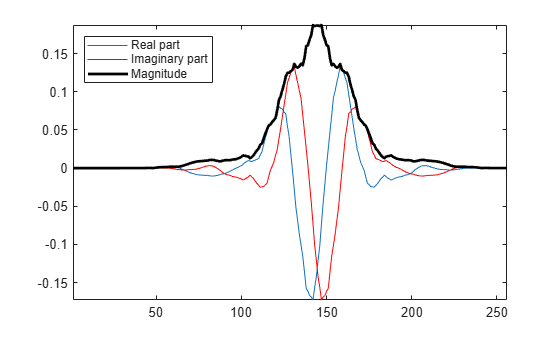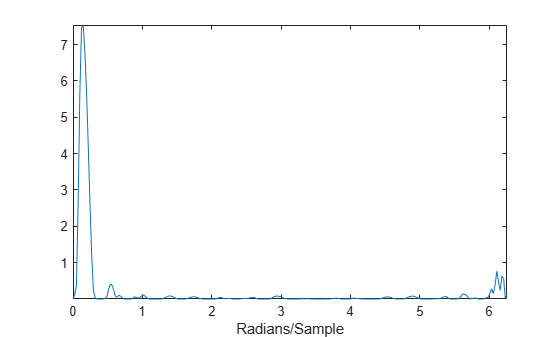## Analytic Wavelets Using the Dual-Tree Wavelet Transform

This example shows how to create approximately analytic wavelets using the dual-tree complex wavelet transform. The example demonstrates that you cannot arbitrarily choose the analysis (decomposition) and synthesis (reconstruction) filters to obtain an approximately analytic wavelet. The FIR filters in the two filter banks must be carefully constructed in order to obtain an approximately analytic wavelet transform and derive the benefits of the dual-tree transform.

Obtain the lowpass and highpass analysis filters.

`DF = dtfilters('dtf1');`

`DF` is a 1-by-2 cell array of $N$-by-2 matrices containing the first-stage lowpass and highpass filters, `DF{1}`, and the lowpass and highpass filters for subsequent stages, `DF{2}`.

Create the zero signal 256 samples in length. Obtain two dual-tree transforms of the zero signal down to level 5.

```x = zeros(256,1); wt1 = dddtree('cplxdt',x,5,DF{1},DF{2}); wt2 = dddtree('cplxdt',x,5,DF{1},DF{2});```

Set a single level-five detail coefficient in each of the two trees to 1 and invert the transform to obtain the wavelets.

```wt1.cfs{5}(5,1,1) = 1; wt2.cfs{5}(5,1,2) = 1; wav1 = idddtree(wt1); wav2 = idddtree(wt2);```

Form the complex wavelet using the first tree as the real part and the second tree as the imaginary part. Plot the real and imaginary parts of the wavelet.

```analwav = wav1+1i*wav2; plot(real(analwav)); hold on; plot(imag(analwav),'r') plot(abs(analwav),'k','linewidth',2) axis tight; legend('Real part','Imaginary part','Magnitude','Location','Northwest');```Fourier transform the analytic wavelet and plot the magnitude.

```zdft = fft(analwav); domega = (2*pi)/length(analwav); omega = 0:domega:(2*pi)-domega; clf; plot(omega,abs(zdft)) xlabel('Radians/sample');```The Fourier transform of the wavelet has support on essentially only half of the frequency axis.

Repeat the preceding procedure with two arbitrarily chosen orthogonal wavelets, `'db4'` and `'sym4'`.

```[LoD1,HiD1] = wfilters('db4'); [LoD2, HiD2] = wfilters('sym4'); df = {[LoD1' HiD1'],[LoD2',HiD2']}; wt1 = dddtree('cplxdt',x,5,df,df); wt2 = dddtree('cplxdt',x,5,df,df); wt1.cfs{5}(5,1,1) = 1; wt2.cfs{5}(5,1,2) = 1; wav1 = idddtree(wt1); wav2 = idddtree(wt2); analwav = wav1+1i*wav2; zdft = fft(analwav); domega = (2*pi)/length(analwav); omega = 0:domega:(2*pi)-domega; clf; plot(omega,abs(zdft))```Using arbitrary orthogonal wavelets in the two trees does not result in approximately analytic wavelets. The Fourier transform of the resulting wavelet has support over the entire frequency axis.

##### SupportGet trial now